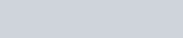Whenever a reaction between an oxidising agent and a reducing agent is carried out,
Question:

Whenever a reaction between an oxidising agent and a reducing agent is carried out, a compound of lower oxidation state is formed if the reducing agent is in excess and a compound of higher oxidation state is formed if the oxidising agent is in excess. Justify this statement giving three illustrations.

Solution:

Whenever a reaction between an oxidising agent and a reducing agent is carried out, a compound of lower oxidation state is formed if the reducing agent is in excess and a compound of higher oxidation state is formed if the oxidising agent is in excess. This can be illustrated as follows:

(i) $P_{4}$ and $F_{2}$ are reducing and oxidising agents respectively.

If an excess of $P_{4}$ is treated with $F_{2}$, then $P F_{3}$ will be produced, wherein the oxidation number (O.N.) of $P$ is $+3$.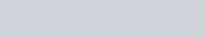However, if $\mathrm{P}_{4}$ is treated with an excess of $\mathrm{F}_{2}$, then $\mathrm{PF}_{5}$ will be produced, wherein the $\mathrm{O} . \mathrm{N}$. of $\mathrm{P}$ is $+5$.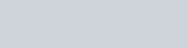(ii) $\mathrm{K}$ acts as a reducing agent, whereas $\mathrm{O}_{2}$ is an oxidising agent.

If an excess of $\mathrm{K}$ reacts with $\mathrm{O}_{2}$, then $\mathrm{K}_{2} \mathrm{O}$ will be formed, wherein the $\mathrm{O} \cdot \mathrm{N}$. of $\mathrm{O}$ is $-2$.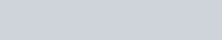However, if $\mathrm{K}$ reacts with an excess of $\mathrm{O}_{2}$, then $\mathrm{K}_{2} \mathrm{O}_{2}$ will be formed, wherein the $\mathrm{O} . \mathrm{N} .$ of $\mathrm{O}$ is $-1$.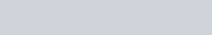(iii)C is a reducing agent, while $\mathrm{O}_{2}$ acts as an oxidising agent.

If an excess of $\mathrm{C}$ is burnt in the presence of insufficient amount of $\mathrm{O}_{2}$, then $\mathrm{CO}$ will be produced, wherein the $\mathrm{O} \cdot \mathrm{N} \cdot$ of $\mathrm{C}$ is $+2$.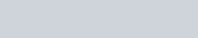On the other hand, if $\mathrm{C}$ is burnt in an excess of $\mathrm{O}_{2}$, then $\mathrm{CO}_{2}$ will be produced, wherein the O.N. of $\mathrm{C}$ is $+4$.